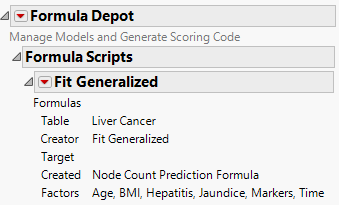Publication date: 11/10/2021

#Example of Formula Depot

The Liver Cancer.jmp sample data table contains data on the severity of liver cancer in patients when they entered a study. The file also contains a number of table scripts for models. This example uses these scripts to generate models to demonstrate the Formula Depot.

1. Select Help > Sample Data Library and open Liver Cancer.jmp.

2. Click the green triangle next to the Lasso Poisson, Validation Column script.

3. Click the red triangle next to Poisson Adaptive Lasso with Validation Column and select Save Columns > Publish Prediction Formula.

This option opens a Formula Depot that contains the prediction formula for the Fit Generalized model.

4. To add the prediction formula to the data table, select Run Script from the Fit Generalized - Node Count red triangle in the Formula Depot.

Note: The result of step 3 and step 4 can be obtained using the Save Columns > Save Prediction Formula at step 3. However, then the prediction formula is not part of the Formula Depot

5. To generate scoring code for use outside of JMP select a code type from the Fit Generalized - Node Count red triangle. A script window appears containing the code. See Generating Scoring Code from the Formula Depot Platform.

6. To save the Formula Depot, select File > Save.

Note: The Formula Depot is saved as a JMP Report File (*.jrp).

Figure 13.2 Formula Depot with a Generalized Model